SSC CGL Previous Year Questions: Geometry- 2

# SSC CGL Previous Year Questions: Geometry- 2 - SSC CGL

Test Description

## 30 Questions MCQ Test SSC CGL (Tier - 1) - Previous Year Papers (Topic Wise) - SSC CGL Previous Year Questions: Geometry- 2

SSC CGL Previous Year Questions: Geometry- 2 for SSC CGL 2023 is part of SSC CGL (Tier - 1) - Previous Year Papers (Topic Wise) preparation. The SSC CGL Previous Year Questions: Geometry- 2 questions and answers have been prepared according to the SSC CGL exam syllabus.The SSC CGL Previous Year Questions: Geometry- 2 MCQs are made for SSC CGL 2023 Exam. Find important definitions, questions, notes, meanings, examples, exercises, MCQs and online tests for SSC CGL Previous Year Questions: Geometry- 2 below.
Solutions of SSC CGL Previous Year Questions: Geometry- 2 questions in English are available as part of our SSC CGL (Tier - 1) - Previous Year Papers (Topic Wise) for SSC CGL & SSC CGL Previous Year Questions: Geometry- 2 solutions in Hindi for SSC CGL (Tier - 1) - Previous Year Papers (Topic Wise) course. Download more important topics, notes, lectures and mock test series for SSC CGL Exam by signing up for free. Attempt SSC CGL Previous Year Questions: Geometry- 2 | 30 questions in 30 minutes | Mock test for SSC CGL preparation | Free important questions MCQ to study SSC CGL (Tier - 1) - Previous Year Papers (Topic Wise) for SSC CGL Exam | Download free PDF with solutions
 1 Crore+ students have signed up on EduRev. Have you?
SSC CGL Previous Year Questions: Geometry- 2 - Question 1

### In ΔPQR, ∠P : ∠Q : ∠R = 2 : 2 : 5. A line parallel to QR is drawn which touches PQ and PR at A and B respectively.What is the value of ∠PBA - ∠PAB?         (SSC Sub. Ins. 2017)

Detailed Solution for SSC CGL Previous Year Questions: Geometry- 2 - Question 1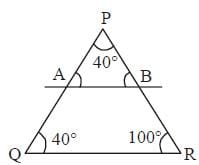In DPQR angles are in the ratio 2:2:5. So, sum of the angles 2x + 2x + 5x = 180°
9x = 180°
2x = 180°
x = 20°
∴ angles are 40°, 40°, 100°.
∵ AB || QR
∴and ∠P = 40°, ∠Q = 40°, ∠R = 100°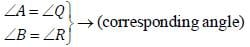∴ Difference ∠PBA and ∠PAB = (100 – 40) = 60°

SSC CGL Previous Year Questions: Geometry- 2 - Question 2

### Find the sum of interior angles of a dodecagon?       (SSC CHSL 2017)

Detailed Solution for SSC CGL Previous Year Questions: Geometry- 2 - Question 2

A dodecagon is a shape with 12 sides and 12 vertices.
i.e. n = 12
Sum of internal angle of a polygon of n sides
= 2(n – 2) × 90°
Here n = 12
∴ Sum of internal angles = 2(12 – 2) × 90° = 1800°

SSC CGL Previous Year Questions: Geometry- 2 - Question 3

### In the given figure, ABC is a triangle in which, AB = 10 cm, AC = 6 cm and altitude AE = 4 cm. If AD is the diameter of the circum-circle, then what is the length (in cm) of circumradius?        (SSC CGL 2017)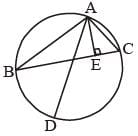Detailed Solution for SSC CGL Previous Year Questions: Geometry- 2 - Question 3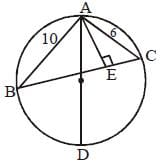From question AB = 10 cm, AC = 6 cm and AE = 4 cm
Now, Area of ΔABC = (1/2) x AE x BC
= (1/2) x 4 x BC
= 2 x BC
By formula, circum radius R =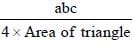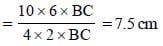SSC CGL Previous Year Questions: Geometry- 2 - Question 4

In the given figure, a smaller circle touches a larger circle at P and passes through its centre O. PR is a chord of length 34 cm, then what is the length (in cm) of PS?        (SSC CGL 2017)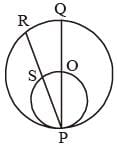Detailed Solution for SSC CGL Previous Year Questions: Geometry- 2 - Question 4

∠PSO is a right angle (angle of semicircle)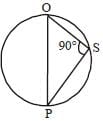Again when OS is perpendicular on chord PR and OS passes through the centre of circle PQR, then it must bisect the chord PR at S.
∴ PS = RS = 17 cm.

SSC CGL Previous Year Questions: Geometry- 2 - Question 5

A chord of length 60 cm is at a distance of 16 cm from the centre of a circle. What is the radius (in cm) of the circle?       (SSC CGL 2017)

Detailed Solution for SSC CGL Previous Year Questions: Geometry- 2 - Question 5

According to question,
AC = 30 cm
OC = 16 cm
From DACO,
AO2 = OC2 + AC2
∴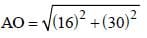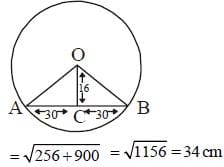∴ radius of circle AO = 34 cm.

SSC CGL Previous Year Questions: Geometry- 2 - Question 6

In ΔABC, ∠BAC = 90° an d AD is drawn perpendicular to BC. If BD = 7 cm and CD = 28 cm, then what is the length (in cm) of AD?        (SSC CGL 2017)

Detailed Solution for SSC CGL Previous Year Questions: Geometry- 2 - Question 6

In DABC
∵ ∠BAC = 90°
∵ (AD)2 = BD × DC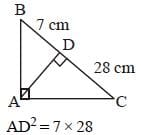∴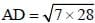SSC CGL Previous Year Questions: Geometry- 2 - Question 7

If there are four lines in a plane, then what cannot be the number of points of intersection of these lines?       (SSC CGL 2017)

Detailed Solution for SSC CGL Previous Year Questions: Geometry- 2 - Question 7

To get a point of intersection, two lines must passes through each other.
Maximum number of point of intersection made by 4 st lines = Number of ways of selecting 2 lines out of 4 lines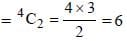Hence, we can't get more than 6 point of intersections from 4st. lines.

SSC CGL Previous Year Questions: Geometry- 2 - Question 8

In triangle PQR, A is the point of intersection of all the altitudes and B is the point of intersection of all the angle bisectors of the triangle. If ∠PBR = 105°, then what is the value of ∠PAR (in degrees)?       (SSC CGL 2017)

Detailed Solution for SSC CGL Previous Year Questions: Geometry- 2 - Question 8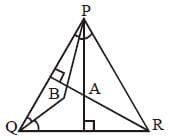From question ∠PBR = 105°
We know that, point of intersection of angle bisector is the in center and point of intersection of altitude is orthocenter.
∠PQR = (105° - 90°) x 2 = 30°
So,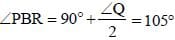Now, ∠PAR + ∠PQR = 180° (orthocenter property)
∠PAR = 180° – 30° = 150°

SSC CGL Previous Year Questions: Geometry- 2 - Question 9

PQR is a right angled triangle in which ∠R = 90°. If  RS ⊥ PQ, PR = 3 cm and RQ = 4 cm, then what is the value of RS (in cm)?      (SSC CGL 2017)

Detailed Solution for SSC CGL Previous Year Questions: Geometry- 2 - Question 9

From question,
PR = 3 cm and
RQ = 4 cm
then,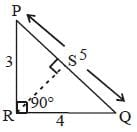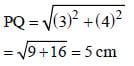Now, ar (ΔPQR) =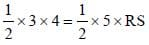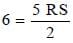∴ RS = 12/5 cm.

SSC CGL Previous Year Questions: Geometry- 2 - Question 10

In triangle ABC, a line is drawn from the vertex A to a point D on BC. If BC = 9 cm and DC = 3 cm, then what is the ratio of the areas of triangle ABD and triangle ADC respectively?       (SSC CGL 2017)

Detailed Solution for SSC CGL Previous Year Questions: Geometry- 2 - Question 10

Let AE is the height of ΔABC.
Then, required ratio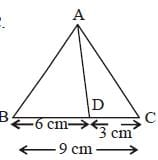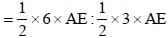⇒ 3 : 1.5
⇒ 2 : 1.

SSC CGL Previous Year Questions: Geometry- 2 - Question 11

In the given figure, O is the centre of the circle and ∠DCE = 45°. If CD = 10√2 cm, then what is the length (in cm) of AC. (CB = BD):       (SSC CGL 2017)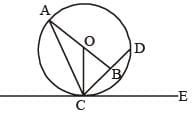Detailed Solution for SSC CGL Previous Year Questions: Geometry- 2 - Question 11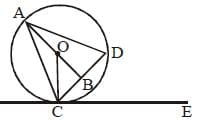According to the question,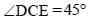From given figure,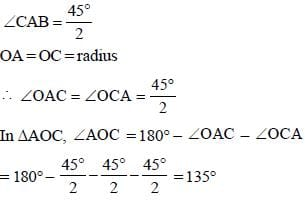Again, ∠AOB = ∠AOC + ∠COB
180° = 135° + ∠COB ⇒ ∠COB = 45°
Now, In DOBC, ∠OBC, ∠OCB = 180° – 90° – 45° = 45°
∴ ∠OB = BC = 5∠2 cm
In ΔOBC, (OC)2 = (OB)2 + (BC)2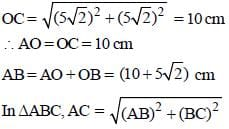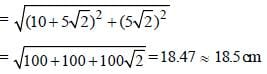SSC CGL Previous Year Questions: Geometry- 2 - Question 12

In the given figure, ABC is a triangle. The bisectors of internal ∠B and external ∠C intersect at D. If ∠BDC = 48°, then what is the value (in degrees) of ∠A?       (SSC CGL 2017)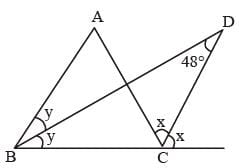Detailed Solution for SSC CGL Previous Year Questions: Geometry- 2 - Question 12

From ΔBCD, x = y + 48°
2x = 24 + 96° ...(i)
From ΔABC,
2x = 2y + ∠CAB ...(ii)
from (i) and (ii)
∠CAB = 96°

SSC CGL Previous Year Questions: Geometry- 2 - Question 13

If length of each side of a rhombus PQRS is 8 cm and ∠PQR = 120°, then what is the length (in cm) of QS?        (SSC CGL 2017)

Detailed Solution for SSC CGL Previous Year Questions: Geometry- 2 - Question 13

∴ ∠QRS = 180° – 120° = 60°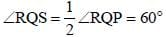∴ ΔRQS is a equlateral triangle.
∴ RQ = QS = 8 cm

SSC CGL Previous Year Questions: Geometry- 2 - Question 14

The perimeter of an isosceles triangle is 32 cm and each of the equal sides is 5/6 times of the base. What is the area (in cm2) of the triangle?        (SSC CGL 2017)

Detailed Solution for SSC CGL Previous Year Questions: Geometry- 2 - Question 14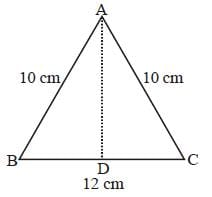Let ΔABC is a isosceles triangle with two equal sides
AB = AC = x cm
then BC = 6/5 x
Now, perimeter AB + BC + AC = 32
x + (6/5)x + x = 32
16x/5 = 32 ⇒ x  = 10 cm
Again let AD is ⊥ on side BC
then BD = 12/2 = 6 cm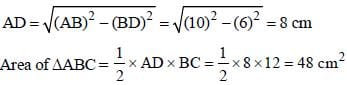SSC CGL Previous Year Questions: Geometry- 2 - Question 15

In ΔPQR, ∠R = 54°, the perpendicular bisector of PQ at S meets QR at T. If ∠TPR = 46°, then what is the value (in degrees) of ∠PQR?       (SSC CGL 2017)

Detailed Solution for SSC CGL Previous Year Questions: Geometry- 2 - Question 15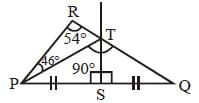From the figure, ∠PTQ = ∠PRT + ∠RPT
∴ ∠PTQ = 54° + 46° = 100° {Exterior angle property}
Now, in ΔPTS and ΔQTQ,
TS = TS (common)
PS = SQ
and ∠PST = ∠QST
∴ PT = TQ ⇒ ∠TPS = ∠TQS
Again in ΔPTQ, ∠TPS + ∠TQS + ∠PTQ = 180°
2∠TQS + 100° = 180°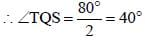Hence, ∠PQR = ∠TQS = 40°

SSC CGL Previous Year Questions: Geometry- 2 - Question 16

In the given figure, ∠QRN  = 40°, ∠PQR = 46° and MN is a tangent at R. What is the value (in degrees) of x, y and z respectively?       (SSC CGL 2017)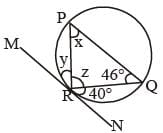Detailed Solution for SSC CGL Previous Year Questions: Geometry- 2 - Question 16

∠MRP = ∠PQR = 46°
∴ ∠y = 46°
And, ∠NRQ = m ∠QPR = 40°
∴∠x = 40°
∠x + ∠z + 46° = 180°
40° + ∠z + 46° = 180°
∴ ∠z = 94°
∴ Value of x, y and z = 40°, 46° and 94°.

SSC CGL Previous Year Questions: Geometry- 2 - Question 17

Two circles of same radius intersect each other at P and Q. If the length of the common chord is 30 cm and distance between the centres of the two circles is 40 cm, then what is the radius (in cm) of the circles?       (SSC CGL 2017)

Detailed Solution for SSC CGL Previous Year Questions: Geometry- 2 - Question 17

∵ ΔPOR = right angle triangle
Let radius of the circle = r cm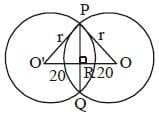PR = 15 cm
RO = 20 cm
∴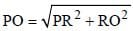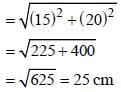SSC CGL Previous Year Questions: Geometry- 2 - Question 18

In the triangle ABC, ∠BAC = 50° and the bisectors of ∠ABC and ∠ACB meets at P. What is the value (in degrees) of ∠BPC?          (SSC CGL 2017)

Detailed Solution for SSC CGL Previous Year Questions: Geometry- 2 - Question 18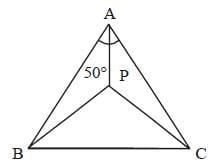∵ ∠BAC = 50°
∵ ∠BPC =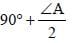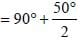= 115°

SSC CGL Previous Year Questions: Geometry- 2 - Question 19

PQR is an equilateral triangle. MN is drawn parallel to QR such that M is on PQ and N is on PR. If PN = 6 cm, then the length of MN is       (SSC CGL 1st Sit. 2016)

Detailed Solution for SSC CGL Previous Year Questions: Geometry- 2 - Question 19

In Given equilateral Δ, MN || QR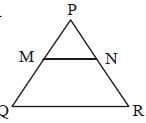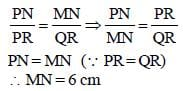SSC CGL Previous Year Questions: Geometry- 2 - Question 20

Two circles touch each other in ternally. The r adius of the smaller circle is 6 cm and the distance between the centre of two circles is 3 cm. The radius of the larger circle is       (SSC CGL 1st Sit. 2016)

Detailed Solution for SSC CGL Previous Year Questions: Geometry- 2 - Question 20

Let two circles with centre O and O' touches each other internally at pont A. radius of smaller circle OA = 6 cm radius of bigger circle O'A = r then OO' = 3 cm
Then radius of bigger circle r = O'A
= O'O + OA = 6 + 3 = 9 cm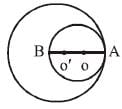SSC CGL Previous Year Questions: Geometry- 2 - Question 21

Number of circles that can be drawn through three noncolinear points is       (SSC CGL 1st Sit. 2016)

Detailed Solution for SSC CGL Previous Year Questions: Geometry- 2 - Question 21

When three points are collinear then, we can not draw any circle that passes through these three points.
Number of circle that passes through three noncollinear points is the same as number of ways of selecting three non-collinear points.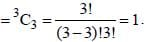SSC CGL Previous Year Questions: Geometry- 2 - Question 22

Which of the following ratios can be the ratio of the sides of a right angled triangle?      (SSC CGL 1st Sit. 2016)

Detailed Solution for SSC CGL Previous Year Questions: Geometry- 2 - Question 22

As only 13, 12 and 5 follows Pythagorous theorem.

SSC CGL Previous Year Questions: Geometry- 2 - Question 23

An arc of 30° in one circle is double an arc in a second circle, the radius of which is three times the radius of the first. Then the angles subtended by the arc of the second circle at its centre is       (SSC CGL 1st Sit. 2016)

Detailed Solution for SSC CGL Previous Year Questions: Geometry- 2 - Question 23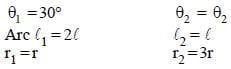Arc length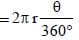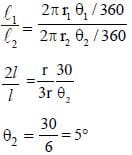SSC CGL Previous Year Questions: Geometry- 2 - Question 24

AB is the diameter of a circle with centre O and P be a point on its circumference, If ∠POA = 120°, then the value of ∠PBO is:        (SSC CGL 1st Sit. 2016)

Detailed Solution for SSC CGL Previous Year Questions: Geometry- 2 - Question 24

∠ POA = 120°
∠ POA = ∠OPB + ∠PBO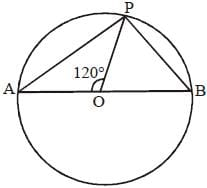∵ OP = OB = radius
∴ ∠OPB = ∠PBO
2 ∠PBO = 120°, ∠PBO = 60°

SSC CGL Previous Year Questions: Geometry- 2 - Question 25

Which of the set of three sides can't form a triangle?      (SSC CGL 1st Sit. 2016)

Detailed Solution for SSC CGL Previous Year Questions: Geometry- 2 - Question 25

5 + 8 < 15
∴ 5, 8, 15 cannot form a triangle.

SSC CGL Previous Year Questions: Geometry- 2 - Question 26

If PA and PB are two tangents to a cirlce with centre O such that ∠APB = 80°. Then, ∠AOP =?       (SSC CGL 1st Sit. 2016)

Detailed Solution for SSC CGL Previous Year Questions: Geometry- 2 - Question 26

∠ APB = 80°
∠ AOB= 180 – 80 = 100°
∠AOP = 100/2 = 50°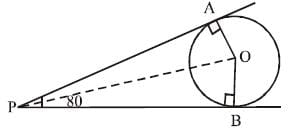SSC CGL Previous Year Questions: Geometry- 2 - Question 27

In a triangle ABC, if ∠A + ∠C = 140° and ∠A + 3∠B = 180°, then ∠A is equal to       (SSC CGL 1st Sit. 2016)

Detailed Solution for SSC CGL Previous Year Questions: Geometry- 2 - Question 27

In ΔABC
∠A + ∠B + ∠C = 180
∠B =180 – [∠A + ∠C)
=180 – 140 = 40°
∠A + 3∠B = 180
∠A =180 – 3 (40)°
= 180 – 120 = 60°

SSC CGL Previous Year Questions: Geometry- 2 - Question 28

The length of the radius of a circle with centre O is 5 cm and the length of the chord AB is 8 cm. The distance of the chord AB from the point O is       (SSC CGL 1st Sit. 2016)

Detailed Solution for SSC CGL Previous Year Questions: Geometry- 2 - Question 28

AB = 8, AD = 4, OA = 5 cm
OD2 = 52 – 42 = 32
OD = 3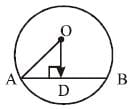SSC CGL Previous Year Questions: Geometry- 2 - Question 29

In ΔPQR, L and M are two points on the sides PQ and PR respectively such that LM II QR. If PL = 2cm; LQ = 6cm and PM = 1.5 cm, then MR (in cm) is       (SSC CGL 1st Sit. 2016)

Detailed Solution for SSC CGL Previous Year Questions: Geometry- 2 - Question 29

By Thales theorem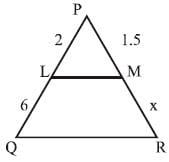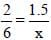x = 4.5 cm

SSC CGL Previous Year Questions: Geometry- 2 - Question 30

X and Y are the mid points of sides AB and AC of a triangle ABC. If  BC + XY = 12 units, then BC – XY is       (SSC CGL 1st Sit. 2016)

Detailed Solution for SSC CGL Previous Year Questions: Geometry- 2 - Question 30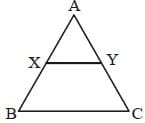In ΔABC ∵X and Y are midpoint of AB and AC
∴ XY = (1/2)BC
2XY = BC
BC + XY = 12
2XY + XY = 12
3XY = 12
XY = 4
BC = 8
Hence, BC – XY = 8 – 4 = 4 unit.

## SSC CGL (Tier - 1) - Previous Year Papers (Topic Wise)

250 tests
Information about SSC CGL Previous Year Questions: Geometry- 2 Page
In this test you can find the Exam questions for SSC CGL Previous Year Questions: Geometry- 2 solved & explained in the simplest way possible. Besides giving Questions and answers for SSC CGL Previous Year Questions: Geometry- 2, EduRev gives you an ample number of Online tests for practice

250 tests